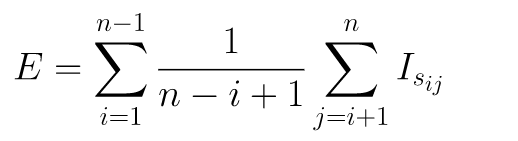# 在Python中，查找排列元素所需的期望洗牌次数的程序

## 程序设计

def is_swapped(i, j, a):
return a[i] < a[j]def expected_shuffle_times(n, target_a):
a = sorted(target_a)
E = 0
for i in range(1, n):
for j in range(i+1, n+1):
if is_swapped(i-1, j-1, target_a):
E += 1/(n-i+1)
return E


if __name__ == '__main__':
a = [5, 7, 3, 6, 2, 1, 8, 4]
n = len(a)
E = expected_shuffle_times(n, a)
print(E)


• 微信订阅
• 回顶